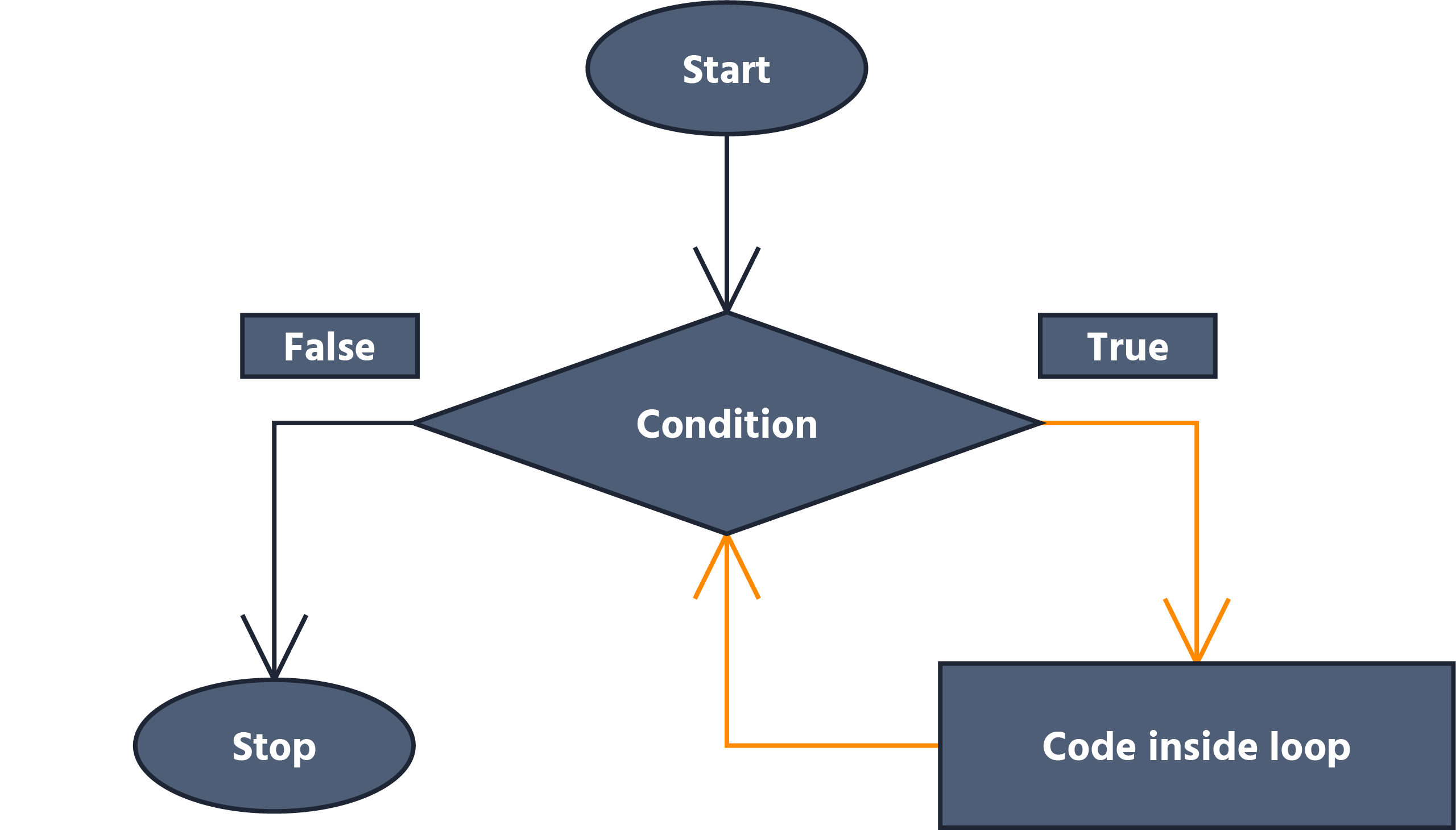Course Content

Java Basics

##While-Loop## While-Loop Syntax

The `while` loop is used to execute a code block repeatedly as long as a specified condition is true.

It has the following structure:Main.javaHere's how the `while` loop works:

• The condition is evaluated before each iteration. If the condition is true, the code block inside the loop is executed. If the condition is false, the loop is terminated, and the program continues with the next statement after the loop;
• The code block inside the loop can contain one or more statements. These statements will be executed repeatedly as long as the condition remains true;
• It's important to ensure that the condition eventually becomes false, or the loop will run indefinitely, causing an infinite loop.

Here's an example to demonstrate the `while` loop:Main.javaIn this code, we have two variables of type `int`. In our loop, we set a condition that reads as follows: as long as the value of variable `a` is not equal to the value of variable `b`, we increment variable `a` and decrement variable `b`. When their values are equal, we terminate the `while-loop`.

Note

The `while` loop is useful when the number of iterations is not known beforehand and depends on a specific condition. It allows you to repeat a code block until the condition is no longer satisfied.

Print the numbers from 1 to 5 using a while loop.Main.java1. 1. Don't forget to create a variable you will increment before using the while-loop.
2. 2. Use `System.out.println()` before the incrementing if you initialized your variable with a value of `1` and after if you initialized with 0.
3. 3. You need to print numbers from 1 to 5 included, be careful about it.
``````
package com.example;

public class Main {
public static void main(String[] args) {
int i = 1;
while (i <= 5) {
System.out.println(i);
i++;
}
}
}
``````Everything was clear?

Section 3. Chapter 4